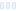# Glycogen Supercompensation Formula

## 1st Phorm

Main info:

Glycogen Supercompensation Formula
1st Phorm
1 scoop
170 Calories
43 g
0 g
0 g

0 g
0 mg
0 g
0 mg
43 g
0 g

Nutrition Facts
For a Serving Size of (g)
How many calories are in Glycogen Supercompensation Formula? Amount of calories in Glycogen Supercompensation Formula: Calories Calories from Fat (%)
% Daily Value *
How much fat is in Glycogen Supercompensation Formula? Amount of fat in Glycogen Supercompensation Formula: Total Fat
How much sodium is in Glycogen Supercompensation Formula? Amount of sodium in Glycogen Supercompensation Formula: Sodium
How much potassium is in Glycogen Supercompensation Formula? Amount of potassium in Glycogen Supercompensation Formula: Potassium
How many carbs are in Glycogen Supercompensation Formula? Amount of carbs in Glycogen Supercompensation Formula: Carbohydrates
How many net carbs are in Glycogen Supercompensation Formula? Amount of net carbs in Glycogen Supercompensation Formula: Net carbs
How much sugar is in Glycogen Supercompensation Formula? Amount of sugar in Glycogen Supercompensation Formula: Sugar
How much protein is in Glycogen Supercompensation Formula? Amount of protein in Glycogen Supercompensation Formula: Protein
Vitamins and minerals
How much Calcium is in Glycogen Supercompensation Formula? Amount of Calcium in Glycogen Supercompensation Formula: Calcium
How much Iron is in Glycogen Supercompensation Formula? Amount of Iron in Glycogen Supercompensation Formula: Iron
Fatty acids
Amino acids
* The Percent Daily Values are based on a 2,000 calorie diet, so your values may change depending on your calorie needs.
Percent calories from...Loading similar foods...
Note: Any items purchased after clicking our Amazon buttons will give us a little referral bonus. If you do click them, thank you!Be cool

- Zen orangeI never skip arm day

- Buff broccoli